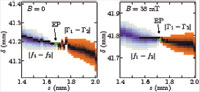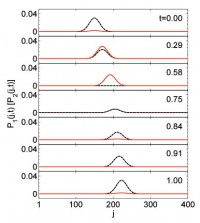July 2011
Mon Tue Wed Thu Fri Sat Sun
« Jun   Aug »
123
45678910
11121314151617
18192021222324
25262728293031

## PT symmetry and spontaneous symmetry breaking in microwave billiards

S. Bittner, B. Dietz, U. Guenther, H. L. Harney, M. Miski-Oglu, A. Richter, F. SchaeferWe demonstrate the presence of parity-time (PT) symmetry for the non-Hermitian two-state Hamiltonian of a dissipative microwave billiard in the vicinity of an exceptional point (EP). The shape of the billiard depends on two parameters. The Hamiltonian is determined from the measured resonance spectrum on a fine grid in the parameter plane. On a curve, which passes through the EP, the Hamiltonian has either real or complex conjugate eigenvalues. An appropriate basis choice reveals its PT symmetry. Spontaneous symmetry breaking occurs at the EP.

http://arxiv.org/abs/1107.4256
Quantum Physics (quant-ph); Mathematical Physics (math-ph)

## Hermitian dynamics in a class of pseudo-Hermitian networks

L. Jin, Z. SongWe investigate the connection between pseudo-Hermitian and Hermitian descriptions for a lattice, which consists of a set of isomorphic pseudo-Hermitian clusters. We show that such non-Hermitian systems can act as Hermitian systems. This is made possible by considering the dynamics of a state involving the superposition of a single eigenmode of each isomorphic clusters. It still holds when multiple eigenmodes are involved when additional restriction on the state is imposed. This Hermitian dynamics is demonstrated for the case of an exactly solvable PT-symmetric ladder system.

http://arxiv.org/abs/1107.4311
Quantum Physics (quant-ph)